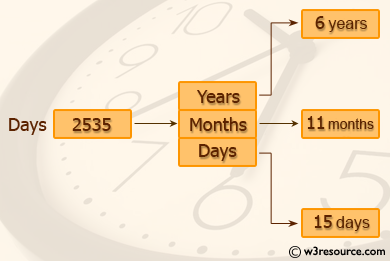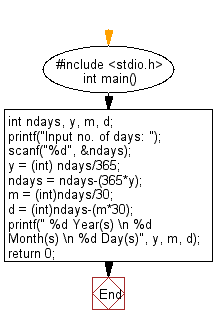﻿ C Program: Convert a given integer to years, months and days - w3resource

# C Exercises: Convert a given integer to years, months and days

## C Basic Declarations and Expressions: Exercise-18 with Solution

Write a C program to convert a given integer (in days) to years, months and days, assuming that all months have 30 days and all years have 365 days.C Code:

``````#include <stdio.h>
int main() {
int ndays, y, m, d; // Declare variables for number of days, years, months, and days

// Prompt user for input number of days and store in 'ndays'
printf("Input no. of days: ");
scanf("%d", &ndays);

// Calculate years, months, and remaining days
y = (int) ndays/365;
ndays = ndays-(365*y);
m = (int)ndays/30;
d = (int)ndays-(m*30);

// Print the result
printf(" %d Year(s) \n %d Month(s) \n %d Day(s)", y, m, d);

return 0;
}
``````

Sample Output:

```Input no. of days: 2535
6 Year(s)
11 Month(s)
15 Day(s)
```

Flowchart:C Programming Code Editor:

What is the difficulty level of this exercise?

Test your Programming skills with w3resource's quiz.

﻿